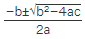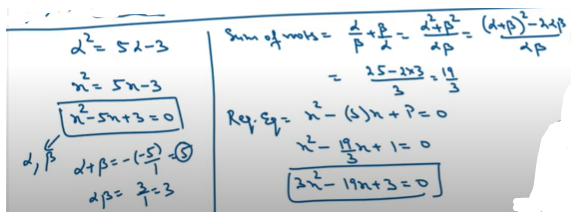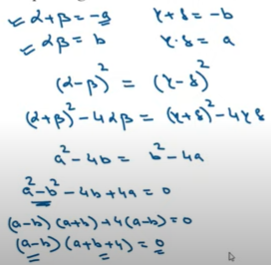• Home

# Quadratic Equation Questions for DU JAT and IPMAT

Author : Palak Khanna

Updated On : June 8, 2023

SHARE

In most of the entrance exams, you will have a mathematics section that includes questions from various concepts and the quadratic equation is one of the essential topics in the Mathematics subject.

It might look complex, but you can solve them in no time if you apply the correct formulas and the right methods.

This post will take you through the essential questions for DU JAT and  IPMAT entrance exams.

Several examples of important questions, solutions, and practice papers are offered in the blog.

Quadratic equations are a type of equation in algebra that can be rearranged in standard form as ax^{2}+bx+c=0 where x represents an unknown, and a, b, and c represent known numbers, and a ≠ 0.

If a = 0, the equation is linear, not quadratic, as there is no ax^2 term.

Examples of the standard form of a quadratic equation (ax² + bx + c = 0) include:

• 6x² + 11x - 35 = 0.
• x² -x - 3 = 0.
• 2x² - 4x - 2 = 0.
• -4x² - 7x +12 = 0.
• 5x² - 2x - 9 = 0.
• 20x² -15x - 10 = 0.

## How to Solve DU JAT Quadratic Equation Questions?

You can solve below questions using various methods; one such is by factorization.

1. Firstly, you need to put all the terms on one side of the equal sign, leaving zero on the other side.
2. Do the factorization.
3. Set each factor equal to zero.
4. Solve each of these equations.For more questions, download the pdf below, which includes questions and solutions. Enhance your preparation for DU JAT by solving these questions and score good marks in the mathematics section.

### Important Formulas to Solve Quadratic Equation Questions for DU JAT and IPMAT

Here is the list of formulas that you can use to solve DU JAT Questions.

• The standard form of a quadratic equation is ax2 + bx + c = 0
• The discriminant of the quadratic equation is D = b- 4ac
• For D > 0 the roots are real and distinct.
• For D = 0 the roots are real and equal.
• For D < 0 the roots do not exist, or the roots are imaginary.
• The formula to find the roots of the quadratic equation is x =• The sum of the roots of a quadratic equation is α + β = -b/a = - Coefficient of x/ Coefficient of x2.
• The product of the Root of the quadratic equation is αβ = c/a = Constant term/ Coefficient of x2
• The quadratic equation having roots α, β, is x2 - (α + β)x + αβ = 0.
• For positive values of a (a > 0), the quadratic expression f(x) = ax+ bx + c has a minimum value at x = -b/2a.
• For negative value of a (a < 0), the quadratic expression f(x) = ax+ bx + c has a maximum value at x = -b/2a.
• For a > 0, the range of the quadratic equation ax2 + bx + c = 0 is [b2 - 4ac/4a, ∞)
• For a < 0, the range of the quadratic equation ax2 + bx + c = 0 is : (∞, -(b2 - 4ac)/4a]

## Important Quadratic Equation Questions and Answers for DU JAT and IPMAT

Here is the list of questions curated from previous year's DU JAT Question Papers. The subject mentor from Supergrads has solved the questions below with a detailed explanation.

Solve these quadratic equations and enhance your preparation for the upcoming DU JAT exam.

1. If 𝛼 ≠ 𝛽 but α 2 = 5α − 3 and β 2 = 5β − 3 then the equation whose roots are 𝛼/𝛽 and 𝛽/𝛼 is

• (a) 3x 2 − 25x +3 = 0
• (b) x 2 + 5𝑥 −3 = 0
• (c) x 2 − 5𝑥 +3 = 0
• (d) 3𝑥 2 − 19𝑥 + 3 = 02. Difference between the corresponding roots of x 2 + ax+ b = 0 and x 2 + bx + 𝑎 = 0 is same and 𝑎 ≠ 𝑏, then

• (a) 𝑎 + 𝑏 + 4 = 0
• (b) 𝑎 + 𝑏 − 4 = 0
• (c) 𝑎 − 𝑏 − 4 = 0
• (d) 𝑎 − 𝑏 + 4 = 03. If p and q are the roots of the equation x2 + px + q = 0 then

• (a) 𝑝 = 1, 𝑞 = −2
• (b) 𝑝 = 0, 𝑞 = 1
• (c) 𝑝 = −2, 𝑞 = 0
• (d) 𝑝 = −2, 𝑞 = 1

4. If a , b , c are distinct positive real numbers and a2 + b 2 + c 2 = 1 then 𝑎𝑏 + 𝑏𝑐 + 𝑐𝑎 is

• (a) less than 1
• (b) equal to 1
• (c) greater than 1
• (d) any real no

5. The value of a for which one root of the quadratic equation (a2 2 − 5a+ 3)x 22 + (3a − 1)x + 2 = 0 is twice as large as the other is

• (a) -2/3
• (b) 1/3
• (c) -1/3
• (d) 2/3

6. If the sum of the roots of the quadratic equation ax2 +bx + c = 0 is equal to the sum of the squares of their reciprocals, then a/c, b/a and c/b are in

• (a) geometric progression
• (b) harmonic progression
• (c) arithmetic-geometric progression
• (d) arithmetic progression

7. Let two numbers have an arithmetic mean nine and geometric mean 4 . Then these numbers are the roots of the quadratic equation

• (a) x 2 + 18𝑥 −16 = 0
• (b) x 2 − 18𝑥 +16 = 0
• (c) x 2 + 18𝑥 +16 = 0
• (d) x 2 − 18𝑥 −16 = 08. If (1 −𝑝) is a root of quadratic equation x2 + 𝑝𝑥 +(1 −𝑝) = 0 then its roots are

• (a) 0, -1
• (b) -1, 1
• (c) 0, 1
• (d) -1, 2

9. If one root of the equation x2+ 𝑝𝑥 + 12 = 0 is 4 while the equation x 2 + 𝑝𝑥 + 𝑞 = 0 has equal roots, then the value of q is

• (a) 3
• (b) 12
• (c) 49/4
• (d) 4

10.  If the roots of the equation x2 −𝑏𝑥 + 𝑐 = 0 be two consecutive integers, then b 2 −4𝑐 equals

• (a) 3
• (b) -2
• (c) 1
• (d) 2

Why should we solve IPMAT Previous Year Papers?Is there any fixed time to take DU JAT Mock Tests?Is DU JAT Exam tough to clear?Which are the best books for DU JAT Exam preparation?Will you provide subject-wise DU JAT Mock Tests?Is Arun Sharma book is good for IPMAT exam?